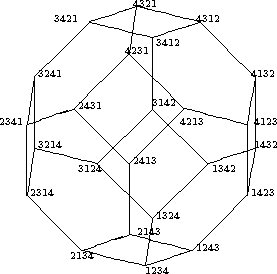Sn as a Coxeter Group

### Sn as a Coxeter Group

Reduced decompositions play a role also in the theory of other groups. The symmetric group Sn is in fact a Coxeter group,

which means that there exists a set of generators si such that the relations are of the particular form (sisj)mij=1, where

mij ÎN, mii=1, mij=mji>1, if i not =j.

In the case of the symmetric group Sn , the Coxeter system of generators is the subset S n of elementary transpositions si=(i,i+1), as we have seen already. Furthermore, this leads us to introduce the weak Bruhat orderon Sn , which is the transitive closure of
p·r : Û l( r)=l( p)+1, and \$ si :r= psi.

The Bruhat order of the symmetric group S 4 is shown in figure. We note that the number of reduced decompositions (and also the set of reduced decompositions) can be obtained by going from the identity upwards in all the possible ways until the permutation in question is reached.The Bruhat order of S 4

We are now approaching a famous result on reduced sequences for the proof of which we need a better knowledge of the set of inversions. In order to describe how I( psi), si ÎS n, arises from I( p), we use that Sn acts on n2 in a canonic way: r(i,j)= ( ri, rj). Keeping this in mind, we easily obtain:

I( psi)= siI( p) È{(i,i+1) } if pi< p(i+1)

and

I( psi)= siI( p) \{(i,i+1) } if pi> p(i+1).

This yields for the corresponding reduced lengths:

l( psi)= l( p)+1 if pi< p(i+1)

and

l( psi)= l( p)-1 if pi> p(i+1),

in accordance with the Lemma. If wn denotes the permutation [n, ...,1] of maximal length, then

l( wn)=[n choose 2], l( wn r)=[n choose 2]-l( r)=l( rwn).

Proof: Clearly I( wn)= {(i,j) În2 | i<j }, and hence l( wn)=[n choose 2]. Moreover

rwn=[ rn, ..., r1],

so I( rwn)= n2 \I( r), and l( rwn)=[n choose 2]- l( r). Finally we note that

l( wn r)=l(( wn r)-1)=l( r-1 wn) =[n choose 2]-l( r-1)=[n choose 2]-l( r),

which completes the proof.

An expression

p= si1...sil, si ÎS n,

of p in terms of elementary transpositions and minimal l=l( p) was called a reduced decomposition of p. The set of corresponding sequences of indices is indicated as follows:

RS( p):= { (i1,...,il) | p= si1...sil, si ÎSn,l minimal }.

These sequences are called reduced sequences

of p.

 harald.fripertinger "at" uni-graz.at http://www-ang.kfunigraz.ac.at/~fripert/ UNI-Graz Institut für Mathematik UNI-Bayreuth Lehrstuhl II für Mathematik
last changed: January 19, 2005Sn as a Coxeter Group